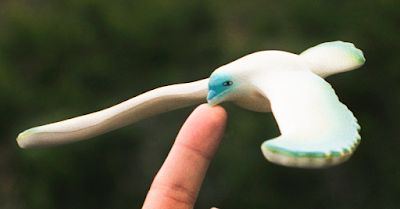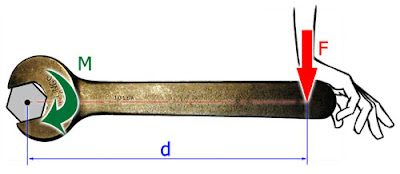## Pages

### 2.3 Centre of gravity, couple and moments.

In the previous section we talked about the forces in equilibrium. Here we'll discuss about the concept of center of gravity, moment.

What is centre of gravity?

-Since any object of concern is not a point mass, but composed of many point masses(atoms), the vector sum of the weights of all the point masses may be taken as acting at a single point. So, basically centre of gravity of a body is the point at which the weight of the body appears to act. The image below shows an example of the principle of centre of gravity to keep balance on the bird.wikipedia.com
Couples: A couple is a pair of forces(same in magnitude but opposite in direction) which tends to produce rotations only.

Torque of couple: It is the product of one of the forces and the perpendicular distance between their lines of action.
$$\tau_{couple}=F_{\perp}\times d$$
Torque of force(moment): The moment or torque of a force is the product of the force and the perpendicular distance between the line of action of the force and the pivot.photocourtesy:learneasy.info

$$\tau_{force}=F_{\perp}\times d$$
As we have seen in the previous section that a body is in equilibrium if there is no resultant force(i.e.$\sum_{i=1}^{n}F_{i}=0$). And so similarly a body is in equilibrium also if no resultant torque is acting on it. (i.e.$\sum_{i=1}^{n}\tau_{i}=0$).

Principle of moments: "For a body in equilibrium under the action of coplanar forces, the no resultant torque condition is due to the fact that clock-wise torque is equal to the anti-clockwise torque about the same point."
$$\sum \tau_{clockwise}=\sum \tau_{anti-clockwise}$$

Ex: Consider a beam(blue color) of length $L$ and weight $W$. And let $F_{1},F_{2}$ be acting at a distance of $\frac{1}{8}L$ and distance of $\frac{1}{4}L$ from its end as shown in the diagram below. Find the $F_{1},F_{2}$ in terms of $W$.

Sol$^{n}$: $F_{1}=(2/5)W$  and $F_{2}=(3/5)W$